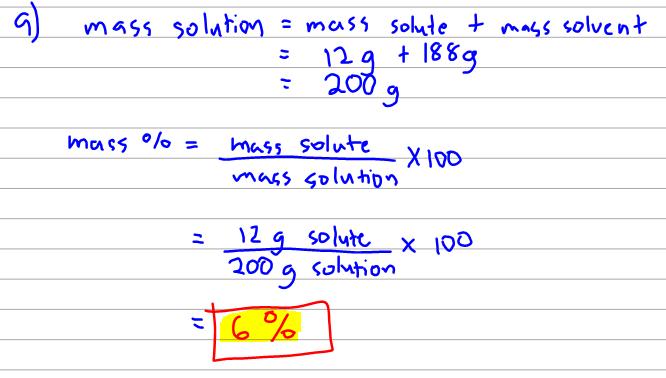# Problem: 12.00 grams of acetic acid HC2H3O2 (Molar mass= 60.00 g/mol) are dissolved in 188.0 grams of water. Density of solution is 1.12g/mL.a. What is the wt/wt % concentration of the solution? b. What is the molarity of this solution?  c. What is the molality of the solution?

###### FREE Expert Solution
80% (106 ratings)
###### FREE Expert Solution

Here we are given the mass of both the solute (acetic acid) and solvent (water) so we can easily get the wt/wt % or also called mass %. Remember that this is the mass of solute over the mass of solution times a hundred so we still have to find the mass of the solution. We can easily get the mass of the solution by adding the masses of the solute and solvent.80% (106 ratings)###### Problem Details

12.00 grams of acetic acid HC2H3O2 (Molar mass= 60.00 g/mol) are dissolved in 188.0 grams of water. Density of solution is 1.12g/mL.

a. What is the wt/wt % concentration of the solution?

b. What is the molarity of this solution?

c. What is the molality of the solution?

What scientific concept do you need to know in order to solve this problem?

Our tutors have indicated that to solve this problem you will need to apply the Mass Percent concept. You can view video lessons to learn Mass Percent. Or if you need more Mass Percent practice, you can also practice Mass Percent practice problems.

What is the difficulty of this problem?

Our tutors rated the difficulty of12.00 grams of acetic acid HC2H3O2 (Molar mass= 60.00 g/mol)...as medium difficulty.

How long does this problem take to solve?

Our expert Chemistry tutor, Dasha took 7 minutes and 20 seconds to solve this problem. You can follow their steps in the video explanation above.

What professor is this problem relevant for?

Based on our data, we think this problem is relevant for Professor Ratliff's class at USF.